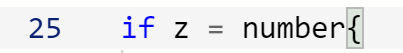← Back to all posts
error cannot assign value of type int to string swift - what am I doing wrong?

print("guess a number that I thought of")
let number = Int.random(in: 0...100)
if z = number{
print("yay")
}

Answered by Coder100 (12858) [earned 5 cycles]
Coder100 (12858)Right here, you forgot an `=`. Remember. `=` is for assignment and `==` is for equality:

``if z == number {``
joshuam6060 (0)

@Coder100
I tried that and it didn't work.

Coder100 (12858)

z has to be of type string @joshuam6060

Coder100 (12858)
``if Int(z) == number {``
Coder100 (12858)

oh right, `z` can be optional.

``if Int(z ?? "0") == number {``